# Difference between revisions of "User talk:WikiSysop"

Test

## Test Copy&Paste HTML

Test-copy-paste Test Test

## Test Asymptote

### Test January 12th 2015

#### Case 1 modified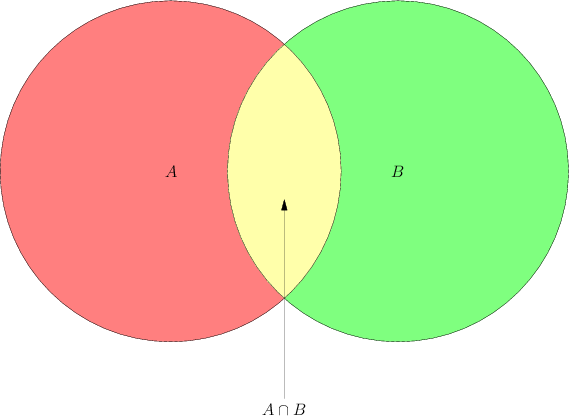### Tests November 1th

#### Case 1 modified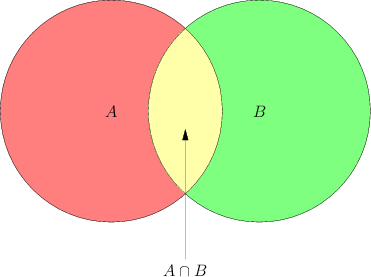#### Case 1### Tests November 17th

#### Case 1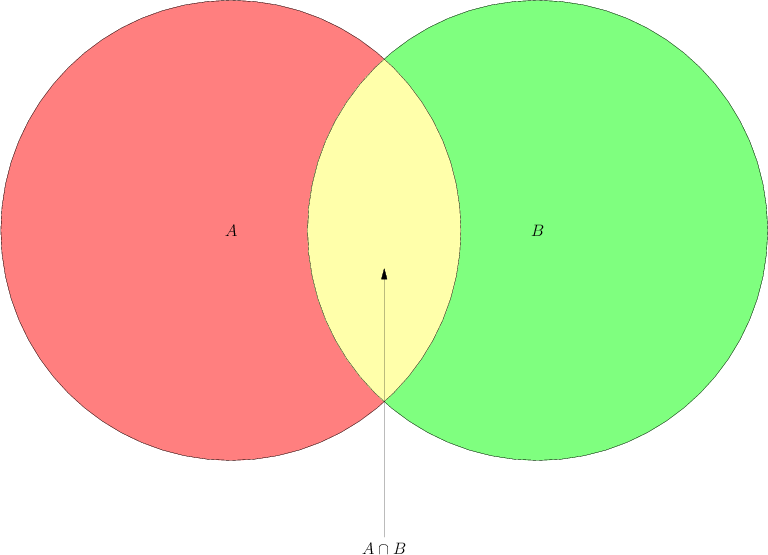### Tests November 4th

#### Case 1#### Case 2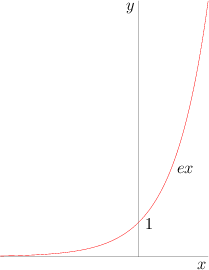#### Case 3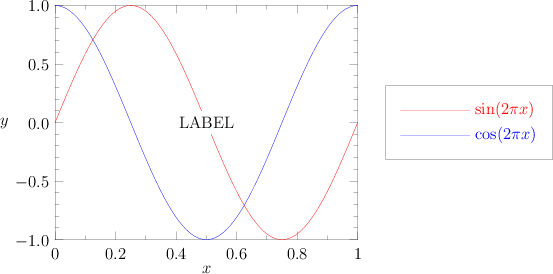### Tests October 27th

#### Case 1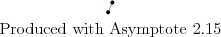#### Case 2#### Case 3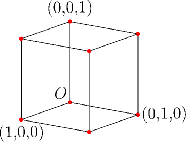#### Case 4#### Case 5

[asy] pair A,B,C,X,Y,Z; A = (0,0); B = (1,0); C = (0.3,0.8); draw(A--B--C--A); X = (B+C)/2; Y = (A+C)/2; Z = (A+B)/2; draw(A--X, red); draw(B--Y,red); draw(C--Z,red); [/asy]

### Previous tests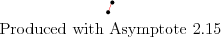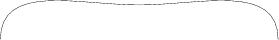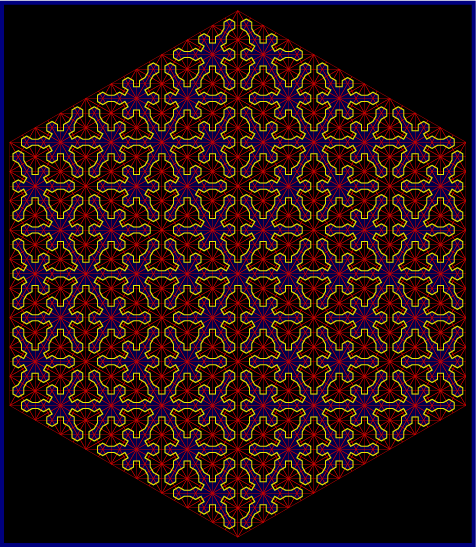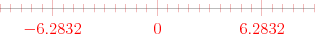## Test Cite Extension

Example: Cite-Extension

## Test MathJax

\begin{align} \dot{x} & = \sigma(y-x) \\ \dot{y} & = \rho x - y - xz \\ \dot{z} & = -\beta z + xy \end{align}

$\frac{1}{(\sqrt{\phi \sqrt{5}}-\phi) e^{\frac25 \pi}} = 1+\frac{e^{-2\pi}} {1+\frac{e^{-4\pi}} {1+\frac{e^{-6\pi}} {1+\frac{e^{-8\pi}} {1+\ldots} } } }$

Some Text $$\frac{1}{(\sqrt{\phi \sqrt{5}}-\phi) e^{\frac25 \pi}} = 1+\frac{e^{-2\pi}} {1+\frac{e^{-4\pi}} {1+\frac{e^{-6\pi}} {1+\frac{e^{-8\pi}} {1+\ldots} } } }$$

Some Text $\frac{1}{(\sqrt{\phi \sqrt{5}}-\phi) e^{\frac25 \pi}} = 1+\frac{e^{-2\pi}} {1+\frac{e^{-4\pi}} {1+\frac{e^{-6\pi}} {1+\frac{e^{-8\pi}} {1+\ldots} } } }$

## Pages A-Z

Alphabetically ordered index of all pages

## Recent Changes

List of previous changes on EOM

.

## Legacy Images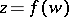How to Cite This Entry:
WikiSysop. Encyclopedia of Mathematics. URL: http://encyclopediaofmath.org/index.php?title=WikiSysop&oldid=41354

Test January 12th 2015